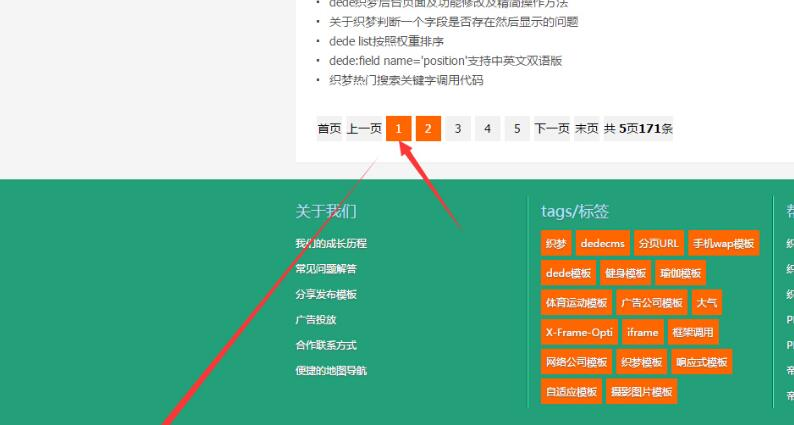>> 更多

### 织梦模板dedecms栏目列表分页链接首页与第一页重复的解决

dede的栏目列表分页链接，仔细观察会发现，在栏目的首页会存在两个内容相同的2个不同url：

1，找到includearc.listview.class.php这个文件，将

1. \$typedir= ereg_replace('{cmspath}',\$GLOBALS['cfg_cmspath'],\$this->Fields['typedir']);

2，找到下面几行代码(就在”//获得上一页和主页的链接”下面)

1.  `1` `if``(\$this->PageNo != 1)`
 `2` `{`
 `3` `\$prepage.=``"
2. 上一页
3. "``;`
 `4` `\$indexpage=”
4. 首页
5. ";`
 `5` `}`

1.  `01` `if``(\$this->PageNo != 1)`
 `02` `{`
 `03` `if``(\$prepagenum==1)`
 `04` `{`
 `05` `\$prepage.=``"
2. 上一页
3. "``;`
 `06` `}`
 `07` `else`
 `08` `{`
 `09` `\$prepage.=``"
4. 上一页
5. "``;`
 `10` `}`
 `11` `\$indexpage=``"
6. 首页
7. "``;`
 `12` `}`

3.找到

1. \$listdd.="<a href='".str_replace("{page}",\$j,\$tnamerule)."'>".\$j."</a> ";

1.  `1` `if``(\$j==1)`
 `2` `{`
 `3` `\$listdd.=``"
2. "``.\$j.``"
3. "``;`
 `4` `}`
 `5` `else`
 `6` `{`
 `7` `\$listdd.=``"
4. "``.\$j.``"
5. "``;`
 `8` `}`